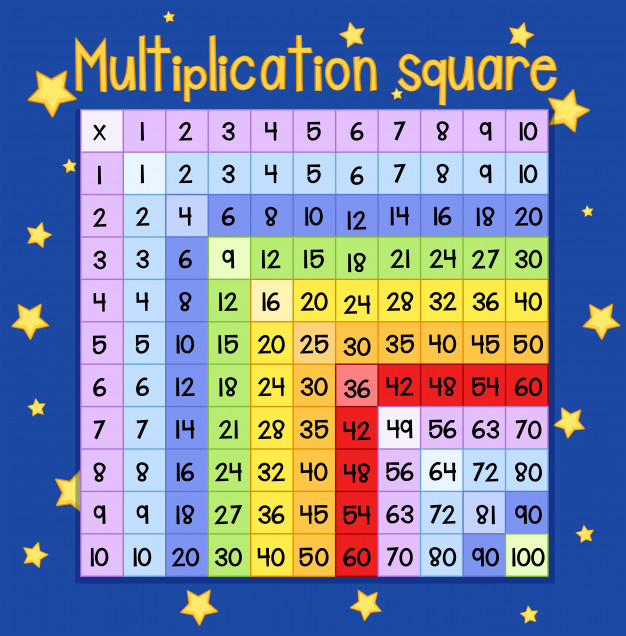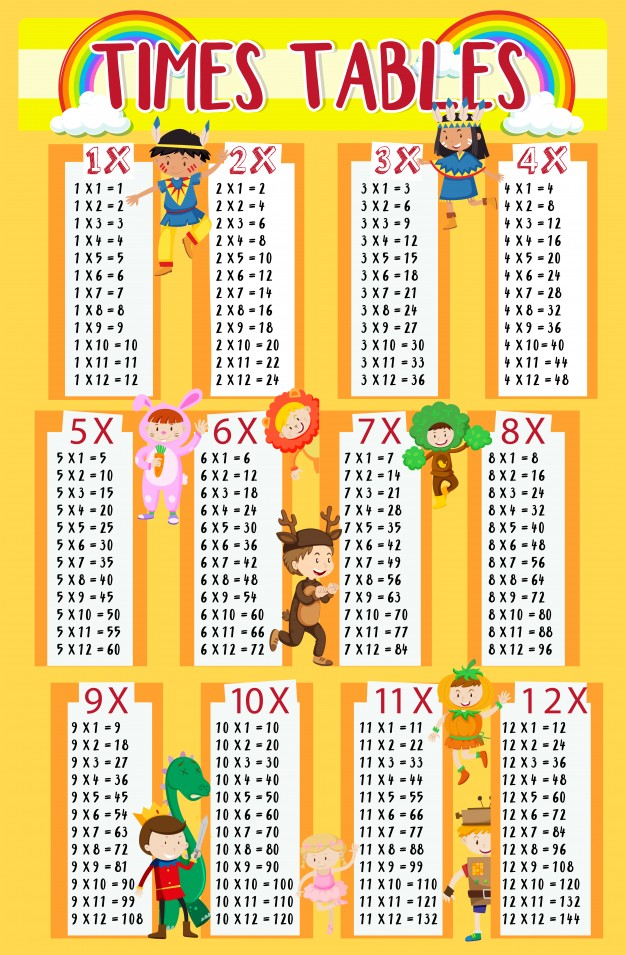Mathematics

# Operations and Algebraic Thinking Grade 3

211 views

 1 Introduction 2 What is Algebraic Thinking? 3 Algebraic thinking for grade 3 4 Operations and Algebraic Thinking Grade 3 Worksheet 5 Summary 6 FAQs 7 External References

15 December 2020

## Introduction

Algebraic thinking includes recognizing and analyzing patterns, studying and representing relationships, making generalizations, and analyzing how things change.

Of course, a facility in using algebraic symbols is an integral part of becoming proficient in applying algebra to solve problems.

But trying to understand abstract symbolism without a foundation in thinking algebraically is likely to lead to frustration and failure. Algebraic thinking can begin when students begin their study of mathematics.

In third grade, students begin to learn multiplication and division. This blog will cover Operations and Algebraic Thinking for grade 3. It talks about basic multiplication, as the product of two different numbers, and Division as splitting a number into parts. Download the PDF below to read on operations and algebraic thinking for Grade 3.

## What is Algebraic Thinking?

Algebraic thinking refers to analyzing and recognizing repeating numeric structures, studying and expressing relations, developing consistent equations, and analyzing changes.

Using symbols and variables also constitutes a big part of algebra, and understanding the basic operations of multiplication and division is essential to becoming proficient in the topic.

## Algebraic thinking for grade 3

### Multiplication

Multiplication is one of the four basic operations; it combines groups of identical sizes. Multiplication can also be expressed as the repeated addition of a specific number a given amount of times.### Properties of Multiplication

• Commutative Property: 5 × 8 = 8 × 5
• Associative Property: 3 × (4 × 5)  = (3 × 4) × 5
 Example 1

7 × 3

Can be expressed as 7 + 7 + 7  = 21 (7 added 3 times)

Or  3 + 3 + 3 + 3 + 3 + 3 + 3 = 21 (3 added 7 times)

 Example 2

4 × 5

Can be expressed as 4 + 4 + 4 + 4 + 4 = 20

Or 5 + 5 + 5 + 5 = 20

 Example 3

7   8

Can be expressed as 7 + 7 + 7 + 7 + 7 + 7 + 7 + 7 = 56

Or 8 + 8 + 8 + 8 + 8 + 8 + 8 = 56Multiplication Tables

### Division

The division is one of four basic operations of mathematics. It refers to the distribution of a quantity into a given number of equal parts. It is the inverse of multiplication. It can also be interpreted as the maximum number of times a specific number can be subtracted from another number.Example 1

8 ÷ 2

8 is to be divided into parts of 2.

8 - 2 - 2 - 2 - 2 = 0 i.e. when 2 is subtracted from  8, 4 times we get zero.

Therefore, 8 ÷ 2 = 4, i.e., 8 can be divided into 4 equal parts of 2 each.

 Example 2

15 ÷ 3

15 is to be divided into parts of 3.

15 - 3 - 3 - 3 - 3 - 3 = 0 i.e. when 3 is subtracted from  15, 5 times we get zero.

Therefore, 15 ÷ 3 = 5, i.e., 15, can be divided into 5 equal parts of 3 each.Pizza divided into 6 equal parts

## Operations and Algebraic Thinking Grade 3 Worksheet

Solve the following questions:

### Multiplication

 1 4 × 7 2 8 × 2 3 6 × 5 4 7 × 7 5 8 × 9 6 10 × 7 7 9 × 9 8 11 × 2 9 12  × 4 10 15 × 5 11 18 × 3 12 20 × 6 13 25 × 2 14 40 × 3 15 45 × 4

### Division

 1 12 ÷ 1 2 4 ÷ 2 3 6 ÷ 3 4 9 ÷ 3 5 12 ÷ 4 6 18 ÷ 6 7 21 ÷ 7 8 35 ÷ 5 9 51 ÷ 3 10 60 ÷ 10 11 80 ÷ 16 12 90 ÷ 90 13 90 ÷ 45 14 100 ÷ 10 15 500 ÷ 100

## Summary

At the earliest grades, young children work with patterns. At an early age, children have a natural love of mathematics, and their curiosity is a strong motivator as they try to describe and extend patterns of shapes, colours, sounds, and eventually letters and numbers.

And at a young age, children can begin to make generalizations about patterns that seem to be the same or different. This kind of categorizing and generalizing is an important developmental step on the journey toward algebraic thinking.

Throughout the elementary grades, patterns are not only an object of study but a tool as well. As students develop their understanding of numbers, they can use patterns in arrays of dots or objects to help them recognize what 6 is or whether 2 is larger than 3.

As they explore and understand addition, subtraction, multiplication, and division, they can look for patterns that help them learn procedures and facts.

This blog talks about two of the fundamental operations in mathematics, it covers the basics and the concept. This blog also has some practice questions and examples. Practising the operations are essential for excelling at them.

Cuemath, a student-friendly mathematics platform, conducts regular Online Live Classes for academics and skill-development and their Mental Math App, on both iOS and Android, is a one-stop solution for kids to develop multiple skills.

Know more about the Cuemath fee here, Cuemath Fee

## What is algebraic thinking?

Algebraic thinking refers to analyzing and recognizing repeating numeric structures, studying and expressing relations, developing consistent equations, and analyzing changes.

## How to learn algebraic thinking?

Practice is very important to master any concept in mathematics. Understanding the concept clearly is also essential, there are games to practice algebraic thinking as well.

## External References

Award-winning math curriculum, FREE for a year